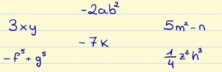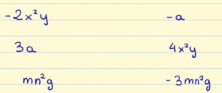Smartick is an advanced online program that teaches kids math and coding in only 15 min. a day

May24

# Learn the Properties of Monomials

In this post, we will look at what monomials are, what their components are and what properties they have.

Let’s start by looking at what a monomial is:

A monomial is a combination of numbers and letters related by only multiplication and the exponents of the letters can only be natural numbers.

For example:

## -5ax 3

Is a monomial because it is a combination of numbers and letters related to each other only by multiplication and the exponent that appears is a natural number.

## -2m 5 + m 3

It is not a monomial because both addition and subtraction appear.

In the following exercise, decide if the algebraic expressions are monomials or not:### The Parts of a Monomial:

• Coefficient: The number the letters are multiplied by.
• Literal part: the letters displayed in the monomial with exponents.
• Degree: The sum of the exponents that the monomial has.
• Variable: Each of the letters in the monomial.

For example, let’s look at the parts of the following monomial:

## -2ab 2

• Coefficient: -2 is the number that accompanies the literal part.
• Literal part: ab 2
• Degree: 1 + 2 = 3. The degree of the monomial is 3.
• Variable: a, b. They are the two letters that appear in the monomial.

### What are two similar monomials?

Two monomials are similar when they have exactly the same literal part.

For example, a similar monomial for one that we’ve seen before, -2ab 2, would be any which had the same literal part: ab 2

Like the monomials: -6ab 2 , 5AB 2 , 18ab 2 , ab 2

Now, I propose that you connect the monomials in the left column with their similar monomials in the right column.And with that, we have finished this post. If you liked this post, share it with your friends and family! And if you want to learn more math, try Smartick for free.